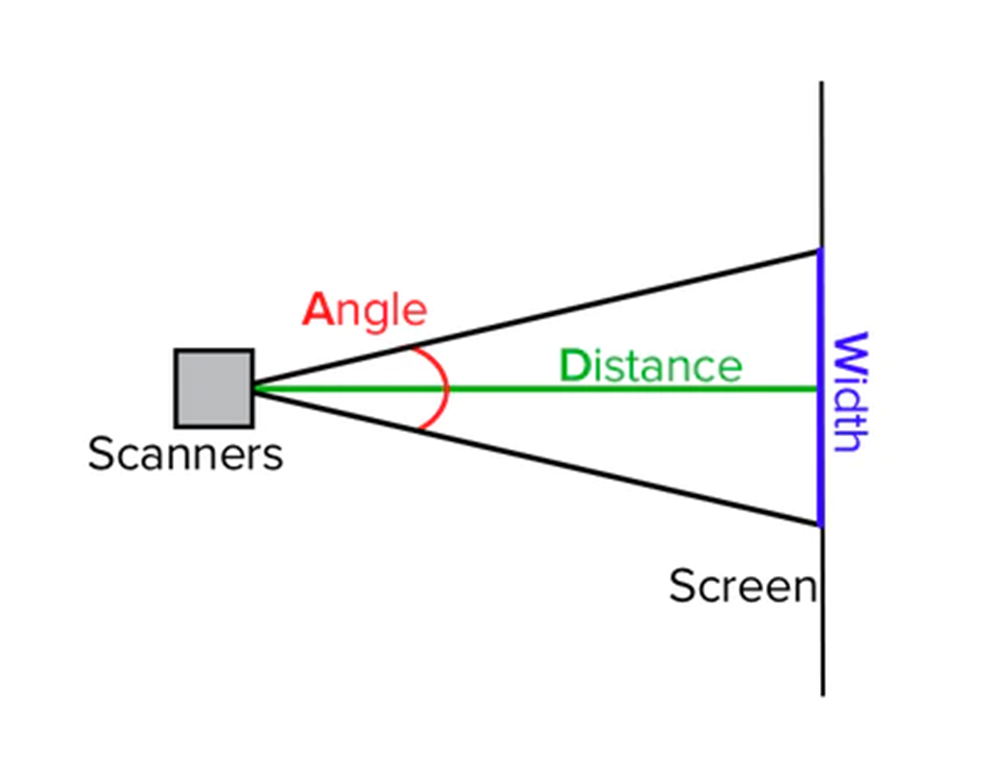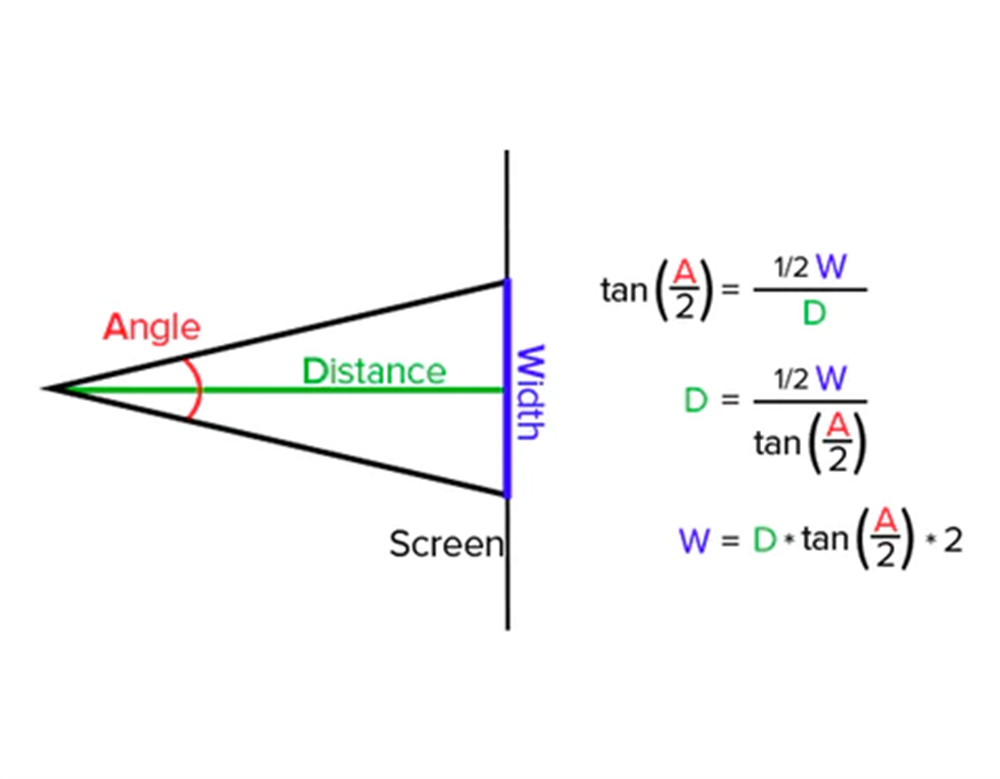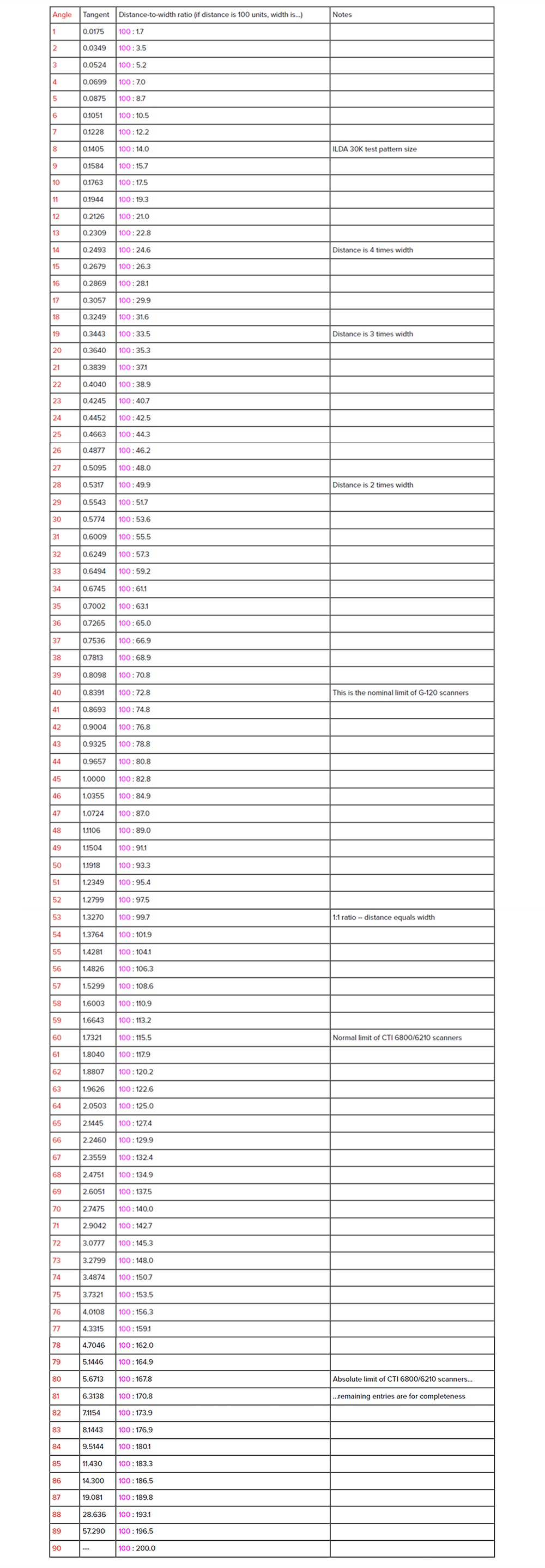# Understanding Scan Angles

by Newfeel Lighting on Sep 19, 2023

• To determine the scanning angle, A, when you have information about the width, W, and the distance, D, follow these steps:
• To determine the distance D when you have knowledge of the scan angle A and the width W, follow these steps:
• To find the width W, knowing the scan angle A and the distance D
• Table of Angle Measurements
In certain scenarios, it becomes essential to determine the scanning angle, screen distance, or image width. The following equations and reference table are designed to simplify this process. To utilize these equations effectively, you must possess knowledge of any two out of the following three parameters:
1. A = Scanning angle in degrees, measured from peak to peak. This is sometimes referred to as the "optical" angle.
2. D = Distance from the scanners to the screen (throw distance).
3. W = Width of the projected image (It's worth noting that the image height will be the same, as both scanners are tuned to the same angle).
It is crucial that both distance and width are expressed in the same units, be it feet, meters, or any other consistent unit of measurement. The choice of units themselves is immaterial. There are two methods available for determining the unknown (third) parameter. The first involves calculations based on the tangent of A (or more precisely, half of A, as the half-angle must be used). The second method involves referring to the distance-to-width ratio provided in the table below. Both approaches yield identical results, so opt for the one that you find most convenient.
For a deeper understanding of how these calculation formulas were derived, please refer to the accompanying diagrams.The projected image width is determined by the scanning angle and the distance to the screen.The origin of the scan angle formulas lies in the realm of right triangles. Specifically, the tangent of the scan angle is defined as the ratio of the length of the side opposite to the angle and the length of the side adjacent to it.A laser scan can be visualized as two right triangles placed in a back-to-back configuration. Therefore, the right triangle formula needs to be adjusted by either doubling or halving values at the relevant stage.
Important Note: While the formulas may entail both multiplication and division by two, these operations should not be regarded as canceling each other out. This discrepancy arises due to the non-linear nature of the tangent function. Consequently, it is essential not to omit any intermediate steps during the calculations.
To determine the scanning angle, A, when you have information about the width, W, and the distance, D, follow these steps:
By Calculation: tan(A / 2) = W / (D * 2)
Let's take an example where W equals 109 meters, and D equals 150 meters. To determine the scanning angle, follow these steps:
1. Begin by doubling D, which results in 300.
2. Now, divide W (109) by 2 times D (300), which equals approximately 0.3633.
3. Refer to the table below to identify the nearest angle with a tangent value of approximately 0.3633. This corresponds to 20 degrees (at 0.3640).
4. Keep in mind that we've found the half-angle of scanning. To determine the actual peak-to-peak angle, double this value, resulting in 40 degrees.
As a result, the desired scan angle, A, is approximately 40 degrees.
Using the table: tablewidth@A = (W * 100) / D
As an illustration, let's consider the values where W is 109 meters and D is 150 meters. To begin, multiply the width W (109) by 100, yielding 10,900. Now, divide this result by the distance D (150), which equals 72.6. Next, refer to the table and scan the "Distance-to-width ratio" column. Find the angle A that corresponds to the ratio closest to 72.6. In this case, it's approximately 40 degrees, where the ratio reads 100:72.8. Consequently, the desired scan angle A is approximately 40 degrees.
To determine the distance D when you have knowledge of the scan angle A and the width W, follow these steps:
By calculation: D = W / (tan(A / 2) * 2)
As an illustration, let's say we have A set at 40 degrees and W equal to 109 meters. To begin, consult the table provided to find the tangent of 40 degrees, which is equivalent to 20 degrees, yielding a value of 0.3640. Now, multiply this value by 2. The equation becomes: W (109) divided by 0.7280, which equals approximately 149.7. Therefore, the required distance D is approximately 150 meters.
Using the table: D = (W / tablewidth@A) * 100
As an illustration, let's consider a scenario where A equals 40 degrees, and the projected image width W is 109 meters. To determine the required distance D, begin by referring to the table provided below and locate the entry for 40 degrees. In the table, you'll find the distance-to-width ratio specified as 100:72.8. Employing the formula, divide the given value for W (109) by 72.8 (the table's width value at the angle A), resulting in 1.497. To obtain the desired distance D, multiply this result by 100, yielding 149.7 meters.
To find the width W, knowing the scan angle A and the distance D
By calculation: W = D * (tan(A / 2) * 2)
As an illustration, let's consider a scenario where A is 40 degrees, and D is 150 meters. To begin, consult the table provided to obtain the tangent value for half of 40 degrees, which is 20 degrees. The tangent of 20 degrees is approximately 0.3640. Now, multiply this value by 2. The resulting equation becomes D (150) multiplied by 0.7280, which equals 109.2. Consequently, the projected image width W is approximately 109 meters.
Using the table: tablewidth@A*W = (D/100)
Let's consider an illustration with A measuring 40 degrees and D being 150 meters. To begin, consult the table presented below and locate the entry for 40 degrees. In the table, the distance-to-width ratio is provided as 100:72.8. Consequently, at angle A, the table indicates a width of 72.8 units.
Now, proceed to divide the value of D, which is 150 meters, by 100, yielding a result of 1.5. Finally, you can calculate the desired width, denoted as W, by multiplying 72.8 by 1.5, resulting in a width of 109 meters.
Table of Angle Measurements